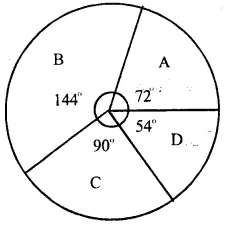## RS Aggarwal Class 8 Solutions Chapter 23 Pie Charts Ex 23A

These Solutions are part of RS Aggarwal Solutions Class 8. Here we have given RS Aggarwal Solutions Class 8 Chapter 23 Pie Charts Ex 23A.

Other Exercises

Question 1.
Solution:
Total expenditure = Rs. 4000 + 5400 + 2800 + 1800 + 400 = Rs. 14400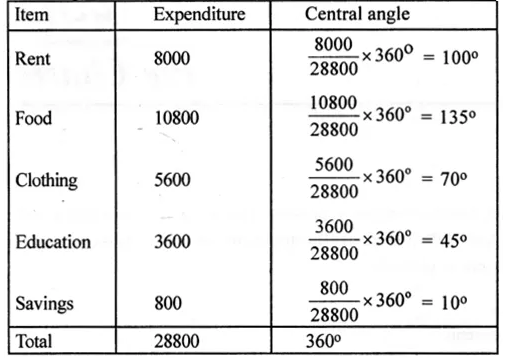Construction of pie chart :
1. Draw a circle of any convenient radius.
3. Staring from this radius, draw sectors of central angle 100°, 135°, 70°, 45° and 10° respectively.
4. Shade these sectors with different colors or designs as shown in the figure.
This is the required pie chart.Question 2.
Solution:
Total number of creatures 900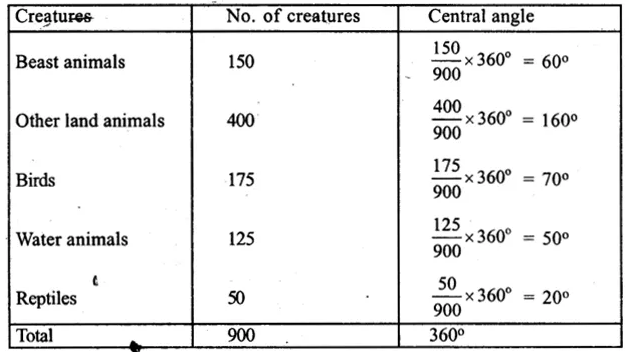(i) Draw a circle with a suitable radius.
(iii) Starting from this radius, draw sectors whose central angles are 60°, 160°, 70°, 50° and 20° respectively.
(iv) Now shade each sector with different colours or designs as shown in the figure.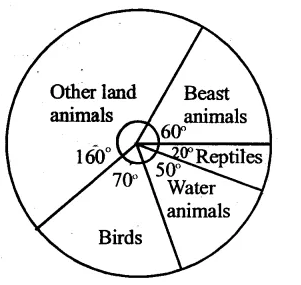Question 3.
Solution:
Total number of students = 350 + 245 + 210 + 175 + 280 = 1260(i) Draw a circle with a suitable radius.
(iii) Starting from this radius draw sectors whose central angles are 100°, 70°, 60°, 50° and 80° respectively.
(iv) Now shade each sector with different colours or designs as shown in the figure.Question 4.
Solution:(i) Draw a circle with a suitable radius.
(iii) Starting from this radius, draw sectors whose actual angles are 105°, 60°, 30°, 120° and 45° respectively.
(iv) Now shade each sector with different colours or design as shown in the figure.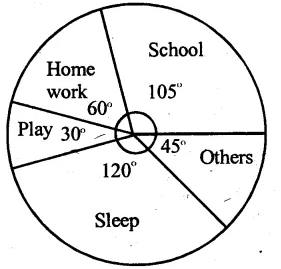Question 5.
Solution:
Here total number of workers = 1080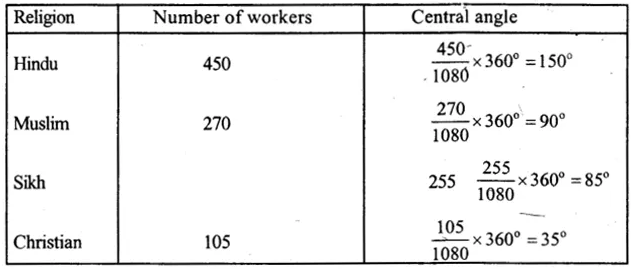Now (i) Draw a circle with a suitable radius
(iii) Starting from this radius, draw sectors whose central angle are 150°, 90°, 85°, 35° respectively.
(iv) Now shade the sectors with different colours or designs as shown in the figure.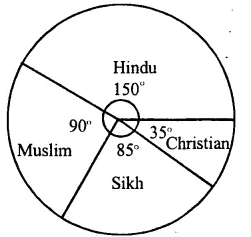Question 6.
Solution:
Total marks obtained by Sudhir
= 105 + 75 + 150 + 120 + 90 = 540(i) Draw a circle with a suitable radius
(iii) Starting from this radius, draw sectors whose central angles are 70°, 50°, 100°, 80° and 60° respectively
(iv) Now shade these sectors with different colours or designs as shown in the figure.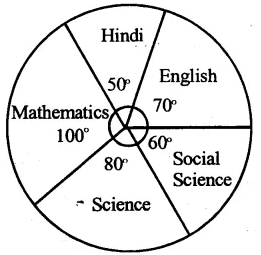Question 7.
Solution:
Total number of fruits = 26 + 30 + 21 + 5 + 8 = 90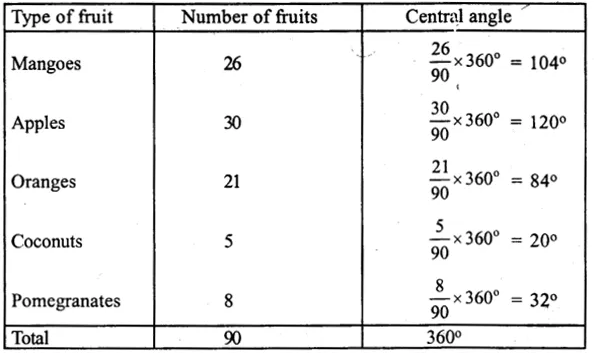(i) Draw a circle with a suitable radius.
(iii) Starting from this radius, draw sectors of central angles 104°, 120°, 84°, 20° and 32° respectively.
(iv) Shade these sectors with different colours or designs as shown in the figure.Question 8.
Solution:
Total number of million of tonnes of food grains = 57 + 76 + 38 + 19 = 190 million of tonnes(i) Draw a circle with suitable radius.
(iii) Starting with this radius, draw sectors of central angles 108°, 144°, 72° and 366 respectively.
(iv) Shade these sectors with different colours or designs as shown in the figure.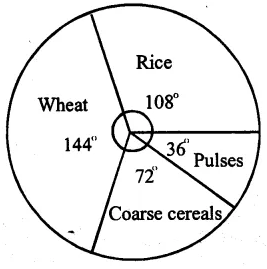Question 9.
Solution:
Total percentage = 25 + 45 + 20 + 10 = 100%(i) Draw a circle with a suitable radius.
(iii) Starting from this radius, draw sectors of central angles 90°, 162°, 72° and 36° respectively.
(iv) Shade these sectors with different colours or designs as shown in the figure.Question 10.
Solution:
Total percentage = 20 + 40 + 25 + 15 = 100%(i) Draw a circle with a suitable radius.